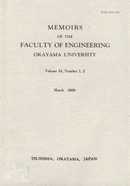Memoirs of the Faculty of Engineering, Okayama University

<Formerly known as>
Memoirs of the School of Engineering, Okayama University

<Availability>
Some items are not available because of decision by its author or publisher.

# A Method of Shape Recognition Using CAD Data and Vertex-Dictionary

We reason the circumstances around the three-dimensional vertex from the information about the intersection point in the two-dimensional image data inputted from a camera on the premise that we use this method as the eye ot the robot. In this method, we use the Vertex-Dictionary. We make the Vertex-Dictionary by calculation from CAD data of object figure (these data are already known) and the position data of the point of view. This dictionary includes the data of position and relations of connect surface etc. about a vertex. We get the data of three-dimensional vertex by comparison the data of two-dimensional intersection point in image data and Vertex-Dictionary. And we get the three-dimensional object by reasoning about the information of circumstances of all vertexes. Then we can recognize the three-dimensional object from image data. In this report, we explain the process to calculate the Vertex-Dictionary and some examples about this method.
ISSN
0475-0071
NCID
AA10699856
NAID